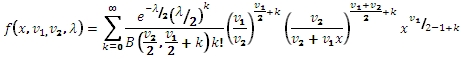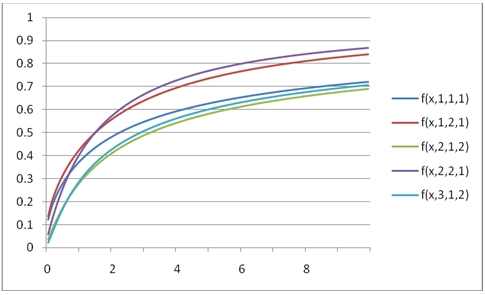# SQL Server non-central F distribution function

NCFCDF

Updated: 31 July 2010

Use NCFCDF to calculate the lower cumulative distribution function of the non-central F distribution.

The formula for the lower cumulative distribution function is:Syntax
SELECT [wctStatistics].[wct].[NCFCDF] (
<@X, float,>
,<@DF1, float,>
,<@DF2, float,>
,<@Lambda, float,>)
Arguments
@X
is the variable to be evaluated. @X is an expression of type float or of a type that implicitly converts to float
@DF1
degrees of freedom. @DF1 is an expression of type float or of a type that implicitly converts to float
@DF2
degrees of freedom. @DF2 is an expression of type float or of a type that implicitly converts to float
@Lambda
is the non-centrality parameter. @Lambda is an expression of type float or of a type that implicitly converts to float
Return Types
float
Remarks
·         @X must be greater than zero (@X > 0).
·         @DF1 must be greater than zero (@DF1 > 0).
·         @DF2 must be greater than zero (@DF2 > 0).
·         @Lambda must be greater than or equal to zero (@Lambda > 0).
Examples
Calculate the lower cumultive distribution function:
SELECT wct.NCFCDF(0.5,3,2,1)
This produces the following result
----------------------
0.210838872156613

(1 row(s) affected)

You can use the SeriesFloat function from the XLeratorDB/math library to generate a dataset which can be pasted into EXCEL to generate a graph of the cumulative distribution function.
SELECT SeriesValue
,wct.NCFCDF(SeriesValue, 1, 1, 1) as [f(x,1,1,1)]
,wct.NCFCDF(SeriesValue, 1, 2, 1) as [f(x,1,2,1)]
,wct.NCFCDF(SeriesValue, 2, 1, 2) as [f(x,2,1,2)]
,wct.NCFCDF(SeriesValue, 2, 2, 1) as [f(x,2,2,1)]
,wct.NCFCDF(SeriesValue, 3, 1, 2) as [f(x,3,1,2)]
FROM wctMath.wct.SeriesFloat(.1, 10, .1,NULL,NULL)

This is an EXCEL-generated graph of the results### SupportCopyright 2008-2023 Westclintech LLC         Privacy Policy        Terms of Service